## Wednesday, 5 October 2011

### HACD optimization

Today, I have updated the HACD library in order to reduce both memory usage and computation complexity (cf. http://sourceforge.net/projects/hacd/).

The new version:

• uses John's Micro Allocator to avoid intensive dynamic memory allocation (thanks John for the great work),
• exploits a simplified version of  an axis-aligned-bounding-volume AABB tree to accelerate dual graph generation (the code is inspired by John's post on this subject, thanks again John :) )
• has an integrated mesh simplification pre-processing step, which makes it possible to handle dense meshes.

To enable mesh decimation, the user specifies the target number of triangles that should be produced before running the convex decomposition. HACD will handle automatically the simplification and the decomposition processes. For the details of the mesh decimation algorithm, have a look at Michael Garland's  webpage http://mgarland.org/research/thesis.html. To turn this feature off just set the parameter targetNTrianglesDecimatedMesh=0.

I have tested the updated HACD algorithm on the 3D model "Bunny", which has 70K triangles. The HACD's parameters were set as follows:
• minNClusters = 12
• maxConcavity = 1000
• ConnectedComponentsDist = 30
• targetNTrianglesDecimatedMesh = 500, 1000, 2000, 4000, 8000 and 16000.

The snapshots of the produced convex decompositions are reported below. The computation times on my machine (Mac Intel Core 2 Duo, 4 GB RAM DDR3) ranged between 3 sec. for 500 triangles and 200 sec. for 16000 triangles (cf. Table 1 for the details).

----------------------------------------
# triangles  Time (sec.)
----------------------------------------
500 2.8
1000 4.6
2000 10
4000 21
8000 70
16000 192
----------------------------------------
Table 1. Computation times for targetNTrianglesDecimatedMesh= 500, 1000, 2000, 4000, 8000 and 16000# triangles after simplification - 500# triangles after simplification - 1000# triangles after simplification - 2000# triangles after simplification - 4000# triangles after simplification - 8000# triangles after simplification - 16000

## Sunday, 2 October 2011

### HACD: Hierarchical Approximate Convex Decomposition

Check out V-HACD 2.0!

I discovered the convex decomposition problem two years ago thanks to a very instructive discussion I had with Stan Melax. At that time, we needed a convex decomposition tool for the game we were working on.  Stan pointed me to John Ratcliff's approximate convex decomposition (ACD) library. Inspired by John's work I developed the HACD algorithm, which was published in a scientific paper at ICIP 2009.

The code I developed back then was  heavily relaying on John's ACD library and Stan's convex-hull implementation (thanks John and Stan :)! ). My implementation was horribly slow and the code was unreadable. When Erwin Coumans contacted me six moths ago asking for an open source implementation of the algorithm, I had no choice than re-coding the method from scratch (i.e. my code was too ugly :) !) . One month later, I published the first version of the HACD library.  Since then, I have been improving it thanks to John's, Erwin's and a lot of other peoples' comments and help. John re-factored the HACD code in order to provide support for user-defined containers (cf. John's HACD). Thanks to Sujeon Kim and Erwin, the HACD library was recently integrated into Bullet.

In this post, I'll try to briefly describe the HACD algorithm and give more details about the implementation. I'll provide also an exhaustive description of the algorithm parameters and discuss how they should be chosen according to the input meshes specificities. By doing so, I hope more people would get interested in the library and would help me improving it.

Why do we need approximate convex decomposition?

Collision detection is essential for realistic physical interactions in video games and computer animation. In order to ensure real-time interactivity with the player/user, video game and 3D modeling software developers usually approximate the 3D models composing the scene (e.g. animated characters, static objects...) by a set of simple convex shapes such as ellipsoids, capsules or convex-hulls. In practice, these simple shapes provide poor approximations for concave surfaces and generate false collision detections.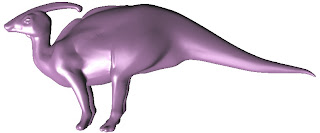Original mesh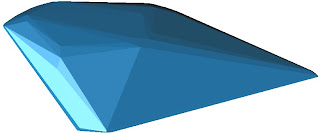Convex-hull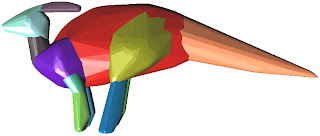Approximate convex decomposition

A second approach consists in computing an exact convex decomposition of a surface S, which consists in partitioning it into a minimal set of convex sub-surfaces. Exact convex decomposition algorithms are NP-hard and non-practical since they produce a high number of clusters. To overcome these limitations, the exact convexity constraint is relaxed and an approximate convex decomposition of S is instead computed. Here, the goal is to determine a partition of the mesh triangles with a minimal number of clusters, while ensuring that each cluster has a concavity lower than a user defined threshold.Exact Convex Decomposition produces 7611 clustersApproximate convex decomposition generates 11 clusters

What is a convex surface?
Definition 1: A set A in a real vector space is convex if any line segment connecting two of its points is contained in it.A convex set in IR2.A non-convex set in IR2.

Let us note that with this definition a sphere (i.e. the two-dimensional spherical surface embedded in IR3)  is a non-convex set in IR3. However, a ball (i.e. three-dimensional shape consisting of a sphere and its interior) is a convex set of IR3.

When dealing with two dimensional surfaces embedded in IR3, the convexity property is re-defined as follows.

Definition 2: A closed surface S in IR3 is convex if the volume it defines is a convex set of IR3.

The definition 2 characterizes only closed surfaces. So, what about open surfaces?

Definition 3: An oriented surface S in IR3 is convex if there is a convex set of IR3 such that is exactly on the boundary of A and the normal of each point of points toward the exterior of A.

The definition 3 do not provide any indication of how to choose the convex set A. A possible choice is to consider the convex-hull of (i.e. the minimal convex set of IR3 containing S), which lead us to this final definition.

Definition 4: An oriented surface S in IR3 is convex if it is exactly on the boundary of its convex-hull CH(S) and the normal of each point of points toward the exterior of CH(S).

With Definition 4, the surface defined by the half of a sphere is convex. The half of a torus is not convex.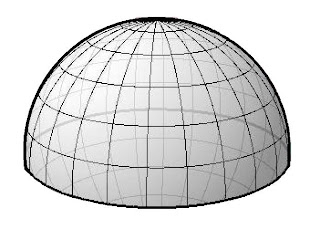Half of a sphere is convex.Half of a torus is not convex.

How to measure concavity?
There is no consensus in the literature on a quantitative concavity measure. In this work, we define the concavity C(S) of a 3D mesh S, as follows:
C(S) = argmax ∥M − P (M )∥, M∈S

where P(M) represents the projection of the point M on the convex-hull CH(S) of S, with respect to the half-ray with origin M and direction normal to the surface S at M.Concavity measure for 3D meshes: distance between M0 and P(M0) (Remark:  S is a non-flat surface. It is represented in 2D to simplify the illustration)

Let us note that the concavity of a convex surface is zero. Intuitively, the more concave a surface the ”farther” it is from its convex-hull. The definition extends directly to open meshes once oriented normals are provided for each vertex.

In the case of a flat mesh (i.e., 2D shape), concavity is measured by computing the square root of the area difference between the convex-hull and the surface:
C_flat(S) = sqrt(Area(CH)-Area(S)).Concavity measure for closed 2D surfaces: the square root of the green area.

Here again, the concavity is zero for convex meshes. The higher C_flat(S) the more concave the surface is. This later definition applies only to closed 2D surfaces, which is enough for HACD decomposition of 3D meshes.

Overview of the HACD algorithm
The HACD algorithm exploits a bottom up approach in order to cluster the mesh triangles while minimizing the concavity of each patch. The algorithm proceeds as follows. First, the dual graph of the mesh is computed. Then its vertices are iteratively clustered by successively applying topological decimation operations, while minimizing a cost function related to the concavity and the aspect ratio of the produced segmentation clusters.

Dual Graph

The dual graph G associated to the mesh S is defined as follows:

• each vertex of G corresponds to a triangle of S,

• two vertices of G are neighbours (i.e., connected by an edge of the dual graph) if and only if their corresponding triangles in S share an edge.Original Mesh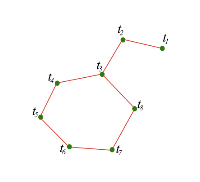Dual Graph
Dual Graph Decimation
Once the dual graph G is computed, the algorithm starts the decimation stage which consists in successively applying halfe-edge collapse decimation operations. Each half- edge collapse operation applied to an edge (v,w), denoted hecol(v, w), merges the two vertices v and w. The vertex w is removed and all its incident edges are connected to v.